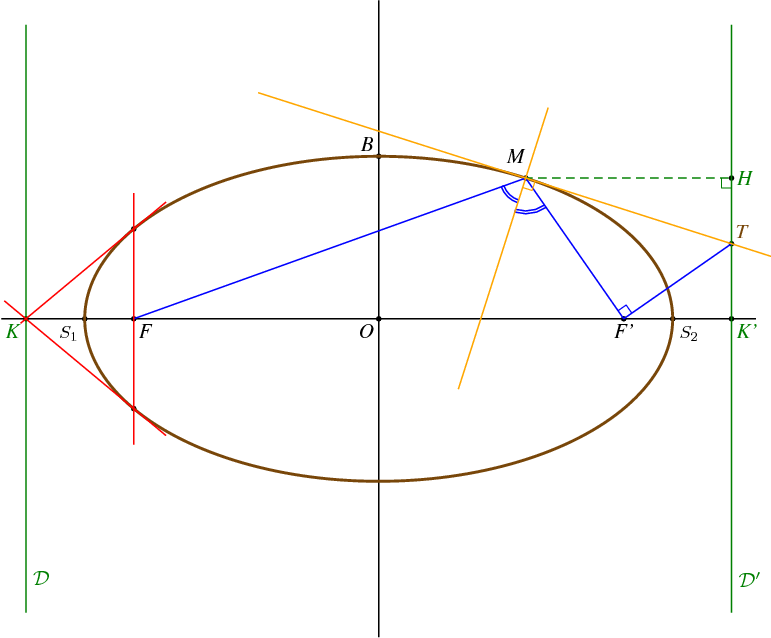Fichier 017.jps — Modifié le 26 Juin 2006 à 14 h 59Source
%% PhS
%%

35 setxunit
-8 8  setxrange
-6.5 6.5 setyrange

%%%%%%%%%%%%%%%%%%%%%%%%%%%%%%%%%%%%%%%%%%%%%%%
%% procédures
%%%%%%%%%%%%%%%%%%%%%%%%%%%%%%%%%%%%%%%%%%%%%%%

/bddroite {
8 dict begin
/kd exch def
/kg exch def
/By exch def
/Bx exch def
/B {Bx By} def
/Ay exch def
/Ax exch def
/A {Ax Ay} def
gsave
[A A B vecteur kg mulv addv A A B vecteur kd mulv addv] ligne
grestore
end
} def

%%%%%%%%%%%%%%%%%%%%%%%%%%%%%%%%%%%%%%%%%%%%%%%
%% definition d'une couleur
%%%%%%%%%%%%%%%%%%%%%%%%%%%%%%%%%%%%%%%%%%%%%%%

/coul1 {120 255 div 70 255 div 9 255 div setrgbcolor} def

%%%%%%%%%%%%%%%%%%%%%%%%%%%%%%%%%%%%%%%%%%%%%%%
%% definition des points
%%%%%%%%%%%%%%%%%%%%%%%%%%%%%%%%%%%%%%%%%%%%%%%

/a {6} def
/c {5} def
/b {a a mul c c mul sub sqrt} def
/e {c a div} def
/Kx {a e div} def
/K {Kx neg 0} def
/K+ {K 0 6 addv} def
/K- {K 0 -6 addv} def
/Kp {Kx 0} def
/Kp+ {Kp 0 6 addv} def
/Kp- {Kp 0 -6 addv} def
/O {0 0} def
/F {c neg 0} def
/Fp {c 0} def
/S1 {a neg 0} def
/S2 {a 0} def
/B {0 b} def

/xdet {settvar t cos a mul} def
/ydet {settvar t sin b mul} def

/Mx {60 xdet} def
/My {60 ydet} def
/M {Mx My} def
/H {M Kp Kp+ orthoproj} def

/bissec {F M Fp bissectrice} def
/bixx {bissec O S2 interdroite}def
/Mbixx {M bixx vecteur} def
/T {bissec M perp  Kp Kp+ interdroite} def
/MT {M T vecteur} def

/theta1 {c neg a div arccos} def
/Fcons1 {theta1 xdet theta1 ydet} def
/Fcons2 {theta1 neg xdet theta1 neg ydet} def

%%%%%%%%%%%%%%%%%%%%%%%%%%%%%%%%%%%%%%%%%%%%%%%
%% traces
%%%%%%%%%%%%%%%%%%%%%%%%%%%%%%%%%%%%%%%%%%%%%%%
1 setlinewidth
[O F Fp S1 S2 B K Kp M T H Fcons1 Fcons2] points

2 setlinewidth
coul1
0 360 settrange
{xdet} {ydet} courbeparam

1 setlinewidth
vert
[K+ K-] ligne
[Kp+ Kp-] ligne
M H Kp angledroit
pointilles
[M H] ligne

bleu
continu
[F M Fp T] ligne
M Fp T angledroit
F M bixx 0.5 (=) tripointarc
bixx M Fp 0.7 (=) tripointarc

orange
continu
M bixx -0.5 1.5 bddroite
M T -1.3 1.8 bddroite
T M bixx angledroit

rouge
Fcons1 Fcons2 -0.2 1.2 bddroite
K Fcons1 -0.05 1.3 bddroite
K Fcons2 -0.2 1.3 bddroite

%%%%%%%%%%%%%%%%%%%%%%%%%%%%%%%%%%%%%%%%%%%%%%%
%% texte
%%%%%%%%%%%%%%%%%%%%%%%%%%%%%%%%%%%%%%%%%%%%%%%

setTimesItalic
noir
(O) O [1.5 dup] dltext
(F) F [1.5 dup] drtext
(F') Fp [1.5 dup] dctext
(B) B [1.5 dup] ultext

<latex>
$S_1$
</latex>
S1 [1.3 dup] dltexlabel

<latex>
$S_2$
</latex>
S2 [1.3 dup] drtexlabel

noir
(M) M -0.2 0.2 addv [1.5 dup] uctext

vert
(K) K [1.5 dup] dltext
(K') Kp [1.5 dup] drtext
(H) H [1.5 dup] crtext

<latex>
$\mathcal{D}$
</latex>
K 0 -5 addv [1.5 dup] drtexlabel

<latex>
$\mathcal{D}'$
</latex>
Kp 0 -5 addv [1.5 dup] drtexlabel

coul1
(T) T [1.5 dup] urtext